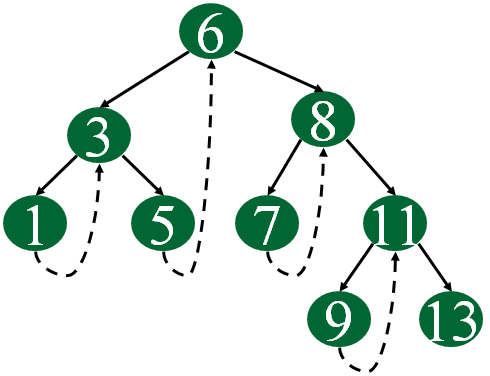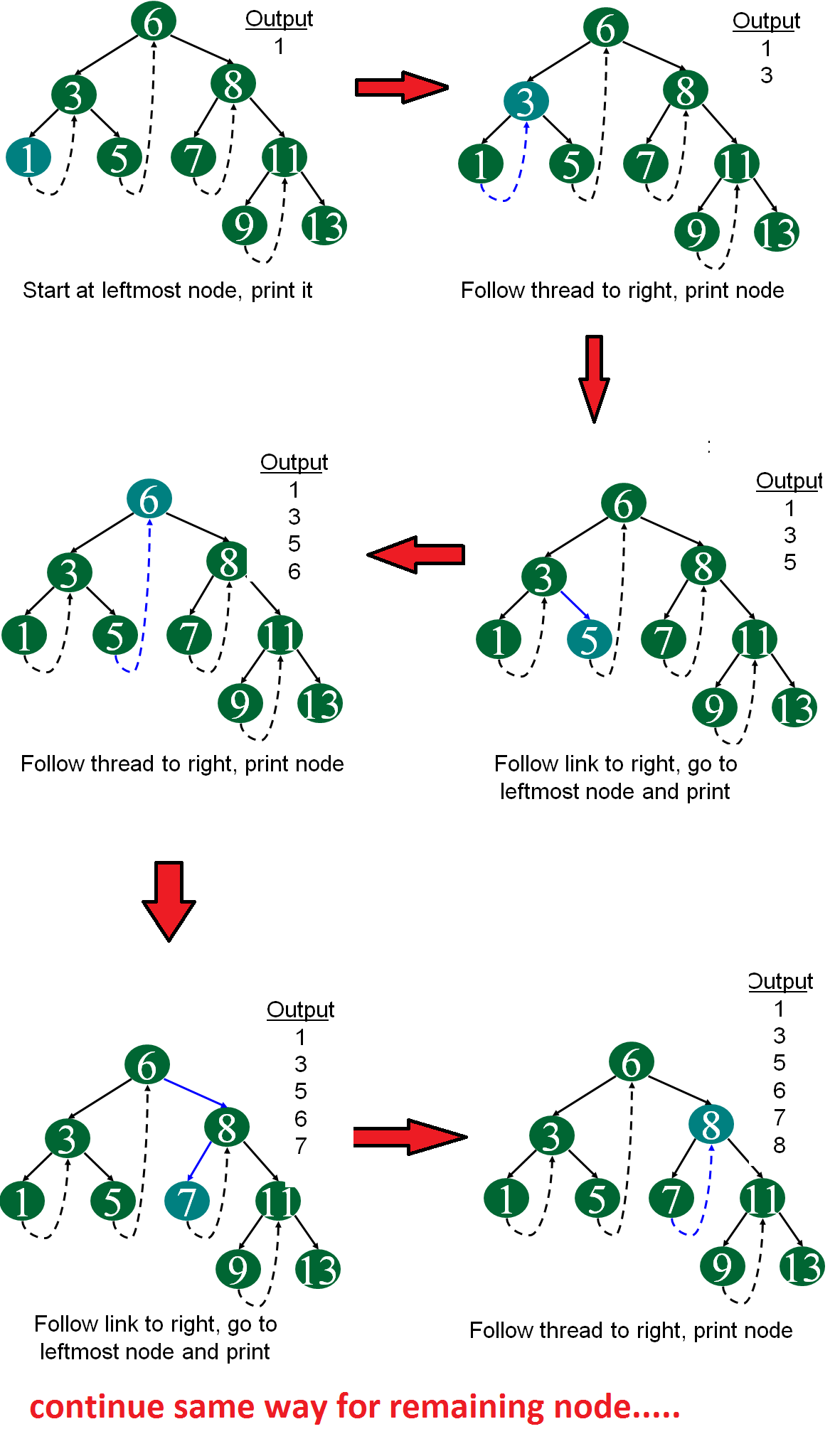• Difficulty Level : Medium
• Last Updated : 12 Apr, 2022

Inorder traversal of a Binary tree can either be done using recursion or with the use of a auxiliary stack. The idea of threaded binary trees is to make inorder traversal faster and do it without stack and without recursion. A binary tree is made threaded by making all right child pointers that would normally be NULL point to the inorder successor of the node (if it exists).
There are two types of threaded binary trees.
Single Threaded: Where a NULL right pointers is made to point to the inorder successor (if successor exists)
Double Threaded: Where both left and right NULL pointers are made to point to inorder predecessor and inorder successor respectively. The predecessor threads are useful for reverse inorder traversal and postorder traversal.
The threads are also useful for fast accessing ancestors of a node.
Following diagram shows an example Single Threaded Binary Tree. The dotted lines represent threads.C representation of a Threaded Node
Following is C representation of a single-threaded node.

## C

 `struct` `Node ` `{` `    ``int` `data;` `    ``struct` `Node *left, *right;` `    ``bool` `rightThread;  ` `}`

Java representation of a Threaded Node

Following is Java representation of a single-threaded node.

## Java

 `static` `class` `Node ` `{` `    ``int` `data;` `    ``Node left, right;` `    ``boolean` `rightThread;  ` `}`   `// This code contributed by aashish1995 `

## Python3

 `class` `Node: ` `    ``def` `__init__(``self``, data,rightThread):` `        ``self``.data ``=` `data;` `        ``self``.left ``=` `None``;` `        ``self``.right ``=` `None``;` `        ``self``.rightThread ``=` `rightThread;`   `# This code is contributed by umadevi9616`

## C#

 `public` `class` `Node ` `{` `   ``public` `int` `data;` `   ``public` `Node left, right;` `   ``public` `bool` `rightThread;  ` `}`   `// This code is contributed by aashish1995`

## Javascript

 ``

Since right pointer is used for two purposes, the boolean variable rightThread is used to indicate whether right pointer points to right child or inorder successor. Similarly, we can add leftThread for a double threaded binary tree.
Following is code for inorder traversal in a threaded binary tree.

## C

 `// Utility function to find leftmost node in a tree rooted` `// with n` `struct` `Node* leftMost(``struct` `Node* n)` `{` `    ``if` `(n == NULL)` `        ``return` `NULL;`   `    ``while` `(n->left != NULL)` `        ``n = n->left;`   `    ``return` `n;` `}`   `// C code to do inorder traversal in a threaded binary tree` `void` `inOrder(``struct` `Node* root)` `{` `    ``struct` `Node* cur = leftMost(root);` `    ``while` `(cur != NULL) {` `        ``printf``(``"%d "``, cur->data);`   `        ``// If this node is a thread node, then go to` `        ``// inorder successor` `        ``if` `(cur->rightThread)` `            ``cur = cur->right;` `        ``else` `// Else go to the leftmost child in right` `             ``// subtree` `            ``cur = leftmost(cur->right);` `    ``}` `}`

## Java

 `// Utility function to find leftmost node in a tree rooted` `// with n` `Node leftMost(Node n)` `{` `    ``if` `(n == ``null``)` `        ``return` `null``;`   `    ``while` `(n.left != ``null``)` `        ``n = n.left;`   `    ``return` `n;` `}`   `// C code to do inorder traversal in a threaded binary tree` `static` `void` `inOrder(Node root)` `{` `    ``Node cur = leftMost(root);` `    ``while` `(cur != ``null``) {` `        ``System.out.printf(``"%d "``, cur.data);`   `        ``// If this node is a thread node, then go to` `        ``// inorder successor` `        ``if` `(cur.rightThread)` `            ``cur = cur.right;` `        ``else` `// Else go to the leftmost child in right` `             ``// subtree` `            ``cur = leftmost(cur.right);` `    ``}` `}`   `// This code contributed by aashish1995`

## Python3

 `# Utility function to find leftmost Node in a tree rooted` `# with n` `def` `leftMost(n):`   `    ``if` `(n ``=``=` `None``):` `        ``return` `None``;`   `    ``while` `(n.left !``=` `None``):` `        ``n ``=` `n.left;`   `    ``return` `n;`     `# C code to do inorder traversal in a threaded binary tree` `def` `inOrder(root):`   `    ``cur ``=` `leftMost(root);` `    ``while` `(cur !``=` `None``):` `        ``print``(cur.data,``" "``);`   `        ``# If this Node is a thread Node, then go to` `        ``# inorder successor` `        ``if` `(cur.rightThread):` `            ``cur ``=` `cur.right;` `        ``else``: ``# Else go to the leftmost child in right` `             ``# subtree` `            ``cur ``=` `leftmost(cur.right);`   `# This code is contributed by Rajput-Ji `

## C#

 `// Utility function to find leftmost node in a tree rooted` `// with n` `Node leftMost(Node n)` `{` `  ``if` `(n == ``null``)` `    ``return` `null``;`   `  ``while` `(n.left != ``null``)` `    ``n = n.left;`   `  ``return` `n;` `}`   `// C code to do inorder traversal in a threaded binary tree` `static` `void` `inOrder(Node root)` `{` `  ``Node cur = leftMost(root);` `  ``while` `(cur != ``null``) ` `  ``{` `    ``Console.Write(``"{0} "``, cur.data);`   `    ``// If this node is a thread node, then go to` `    ``// inorder successor` `    ``if` `(cur.rightThread)` `      ``cur = cur.right;` `    ``else` `// Else go to the leftmost child in right` `      ``// subtree` `      ``cur = leftmost(cur.right);` `  ``}` `}`   `// This code is contributed by gauravrajput1`

## Javascript

 ``

Following diagram demonstrates inorder order traversal using threads.• In this Tree it enables linear traversal of elements.
• It eliminates the use of stack as it perform linear traversal.
• Enables to find parent node without explicit use of parent pointer
• Threaded tree give forward and backward traversal of nodes by in-order fashion
• Nodes contain pointers to in-order predecessor and successor

We will soon be discussing insertion and deletion in threaded binary trees.
Sources: